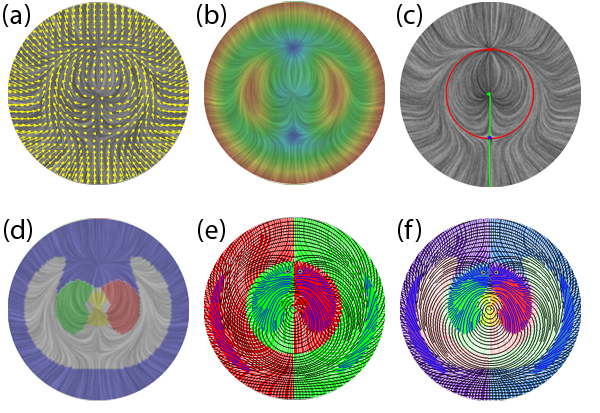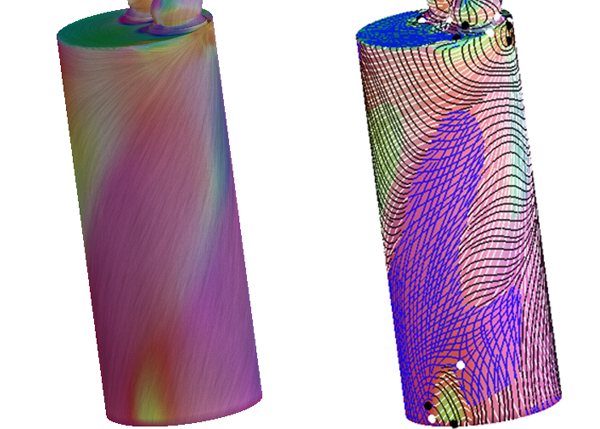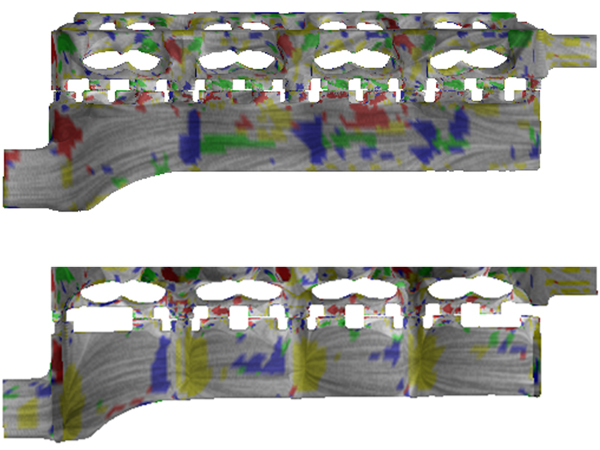Home | CV | Research | Other Projects | Hot List# Asymetric Tensor Analysis for Flow Visualization

Eugene Zhang, Harry Yeh, Zhongzang Lin, and Robert S. Laramee
IEEE Transactions on Visualization and Computer Graphics, vol. 15(1), 2009, pp. 106-122..

Paper (PDF, 4.84Mb).

This material is based upon work supported by the National Science Foundation under Grant No. CCF-0546881.

Any opinions, findings and conclusions or recomendations expressed in this material are those of the author(s) and do not necessarily reflect the views of the National Science Foundation (NSF).

### Abstract

The gradient of a velocity vector field is an asymmetric tensor field which can provide critical insight that is difficult to infer
from traditional trajectory-based vector field visualization techniques. We describe the structures in the eigenvalue and
eigenvector fields of the gradient tensor and how these structures can be used to infer the behaviors of the velocity field that can represent either a 2D compressible flow or the projection of a 3D compressible or incompressible flow onto a two-dimensional manifold.

To illustrate the structures in asymmetric tensor fields, we introduce the notions of eigenvalue manifold and eigenvector manifold. These concepts afford a number of theoretical results that clarify the connections between symmetric and
antisymmetric components in tensor fields. In addition, these manifolds naturally lead to partitions of tensor fields, which we use to design effective visualization strategies. Moreover, we extend eigenvectors continuously into the complex domains which we refer to as pseudo-eigenvectors. We make use of evenly-spaced tensor lines following pseudo-eigenvectors to illustrate the local linearization of tensors everywhere inside complex domains simultaneously.

Both eigenvalue manifold and eigenvector manifold are supported by a tensor reparameterization with physical meaning. This allows us to relate our tensor analysis to physical quantities such as rotation, angular deformation, and dilation, which provide physical interpretation of our tensor-driven vector field analysis in the context of fluid mechanics.

To demonstrate the utility of our approach, we have applied our visualization techniques and interpretation to the study of the Sullivan Vortex as well as computational fluid dynamics simulation data.

### Figures

1. Flow visualization techniques based on arrow plot (a), vector field magnitude (b), vector field topology (c), and our tensor-driven techniques: eigenvalue-based segmentation overlaid with vector field visualization (d), eigenvector analysis where colord indicating real and complex domains (e), and eigenvector analysis overlaid with eigenvalue analysis (f). Tensor-driven flow visualization provides additional and complementary insights of the flow field.2. Diesel engine simulation using an eigenvalue-based method (left) and a hybrid method (right).3. Our technique is applied to the cooling jacket simulation data. Notice the flow and geometry are extremely complex.